Next: Construction of hard-core predicates Up: Further results on pseudo-random Previous: Further results on pseudo-random   Contents

## Hard-Core Predicates and Pseudo-Random Numbers

We have seen several functions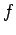with the property thatwas easy to compute butwas difficult. Such anis called a one-way function.16 A predicate is a property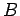which is true or false for any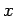. Typical examples might be is an odd number'' or is.'' A hard-core predicate for a functionis a predicate such that
1. there is an efficient algorithm for deciding whetheris true
2. if we are given, there is no efficient algorithm for guessing whetheris true which has a probability much greater thanof being right.
The example we used in the quadratic number generator waswhere we are looking only at thosewhich are squares.

Theorem 32   Ifis a hard-core predicate for, the random number generator in which a seedis chosen at random, giving the sequence of bits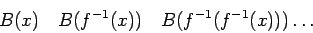satisfies the Next Bit Condition.

As a corollary, the sequence,,...satisfies all efficient tests, using the arguments in the preceding section.

Proof: If there were a program which took as input a sequence of bits and could guess the next bit, we could get a good guess onwithknown by asking the next-bit predictor what would occur next in the sequence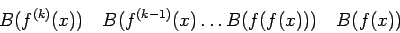(this is really the same proof as in the case of the quadratic generator).

#### Footnotes

... function.16
In later work, we will be defining a one-way function to be such that there is no efficiently computable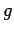with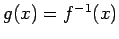for most.Next: Construction of hard-core predicates Up: Further results on pseudo-random Previous: Further results on pseudo-random   Contents
Translated from LaTeX by Scott Sutherland
2002-12-14